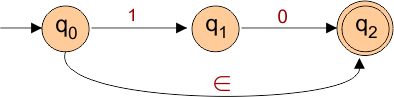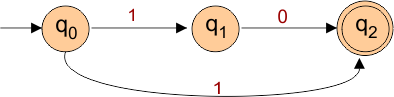Select Page

# Non-Deterministic Finite Automata (NFA)

Non-Deterministic Finite Automata (NDFA or NFA) is an automata which accept all regular languages whether they are finite or infinite. So All DFA are NFA but not vice versa.

Very important In NFA is that,

• Empty String (epsilon) transition is possible.
• No Need to specify the transition of each input from any state.

Formal Definition of NFA

A NFA can be represented by a 5-tuple (Q, ∑, δ, q0, F) where

• Q is a finite set of all states (q0, q1, q2,   … qn). where n is finite number
• is a finite set of symbols called the alphabet. i.e. {0, 1}.
• δ : Q x ∑ → 2Q is a total function called as transition function
• q0 is the initial state from where any input is processed (q0 ∈ Q).
• F is a set of final state/states where F will be subset ( ⊆ ) of Q.

### Example of Non-Deterministic Finite Automata with Epsilon

Example of Non-Deterministic Finite Automata with epsilon is given underThe above NFA can be defined in form of five tuples as-

{Q {q0, q1, q2}, {0, 1}, δ, S {q0}, F {q2} }

where

• {q0, q1, q2} are set of states
• {0, 1} are the set of input alphabets
• δ refers to the transition function
• q0 refers to the the initial state
• {q2} refers to the set of final state

Transition function δ is defined as

• δ (q0, 1) = q1
• δ (q0, ∈) = q2
• δ (q1, 0) = q2

Transition Table for the above Non-Deterministic Finite Automata is

 States / Alphabets 0 1 ∈ q0 – q1 q2 q1 q2 – – q2 – – –

### Example of Non-Deterministic Finite Automata without Epsilon

Following automata is an example of Non-Deterministic Finite Automata without epsilonThe above NFA can be defined in form of five tuples as

{ Q{ q0,q1,q2}, ∑{0,1}, δ, S{q0}, F{q2} }

• set of states are {q0,q1,q2}
• set of input alphabets are {0,1}
• δ refers to the transition function
• initial state is q0
• set of final states are {q2}

Transition function δ can also defined as

• δ (q0, 1) = q1
• δ (q0, 1) = q2
• δ (q1, 0) = q2

Transition Table for the above NDFA is

 States / Alphabets 0 1 q0 – q1, q2 q1 q2 – q2 – –

## Equivalence of DFA and NFA

• Two finite automata accepters are said to be equal in power if the both accepts the same language.
• DFA and NFA both accept the similar languages because DFA and NFA can be constructed for any regular language. So, both exactly equal in power.

Important

• Every NFA is converted into its equivalent DFA.
• Every DFA is a NFA but every NFA is not a DFA.
Help Other’s By Sharing…